# RFEM – Online Manual

Online manuals, introductory examples, tutorials, and other documentation.

# 6.7 Free Line Loads

General description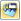A free line load acts as a uniform or linearly variable force along any freely definable line of a surface. No FE nodes are generated along this line.

To apply a free line load, a surface must already be defined.

Line support forces imported from another model by using the Import Support Reactions as Load function (see Figure 8.13) are handled as free line loads.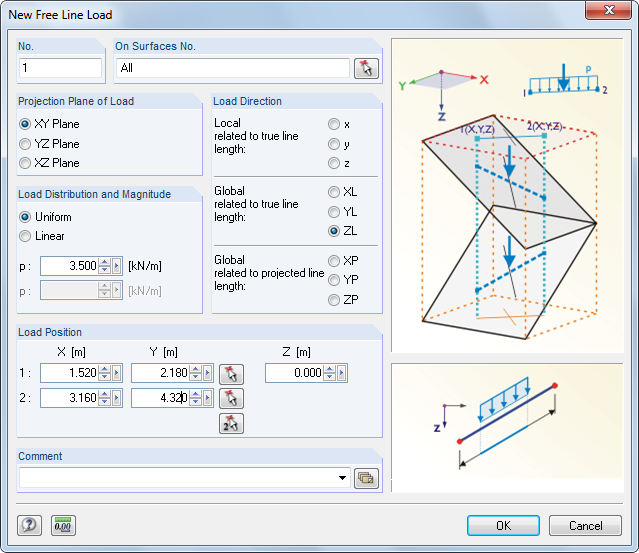Figure 6.36 New Free Line Load dialog box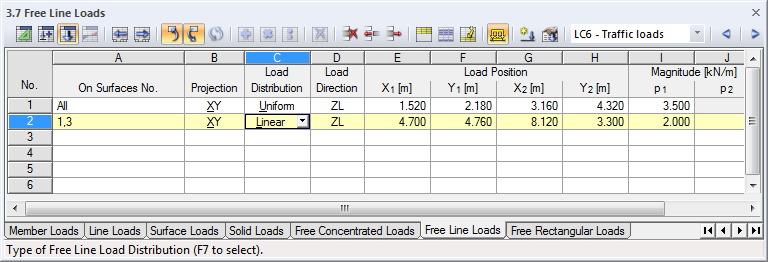Figure 6.37 Table 3.7 Free Line Loads
On Surfaces No.

This text box manages the numbers of the surfaces on which the load acts. You can also select them graphically by using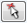.

Starting from both points defined by the Load Position, two imaginary straight lines are "set" perpendicular to the projection plane. If the lines intersect any of the listed surfaces, the load is applied to the connecting line of both intersection points. In this way, it is possible to quickly allocate similar loads to several surfaces.

Projection Plane

The load can be projected onto one of the global planes XY, YZ, or XZ. As described above, two imaginary lines are generated, starting from both load positions and running perpendicular to the projection plane. The start and end points of the free line load are assumed wherever the lines intersect a surface. The projection plane may not be perpendicular to a surface on which the load acts: There are no clear points of intersection with the surface.

Load Distribution

Specify whether a uniform or linearly variable force is applied. In the dialog text box below, you can enter one or two numerical values.

Load Direction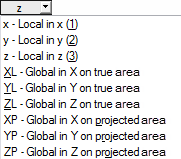The load can act in direction of the local surface axes x, y, z or the global axes X, Y, Z. Loads acting perpendicular to the surface must generally be defined as local in direction z.

If a globally acting load is not perpendicular to the line, then the load impact can be related to different reference lengths:

• Related to true line length
• The load is applied to the entire true line length.
• Related to projected line length in X / Y / Z
• The application length of the load is converted to the projection of the line in one of the directions of the global coordinate system. The projection lengths are illustrated in the dialog graphic in the lower right.
Load Position

Enter the coordinates of the load position into the text boxes. In the dialog box, you can also select the position of the load graphically by using thebutton.

Magnitude

In this table column or text box, enter the numerical value of the line load.